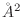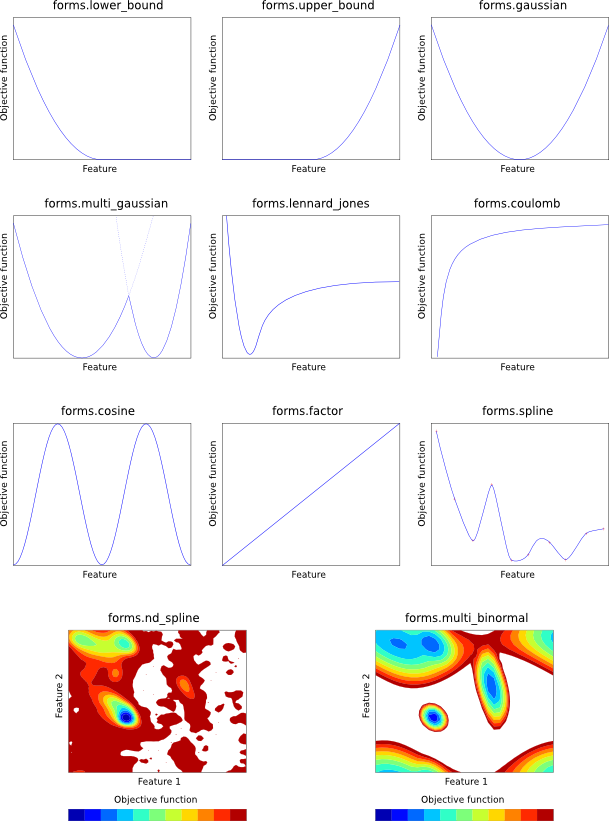Subsections

## Specification of restraints

### Static and dynamic restraints

Dynamic restraints are created on the fly, and currently include:

Dynamic restraints are not written into the restraints file by Restraints.write() (only static restraints are).

Static restraints can be added with the Restraints.add() command, or can be read from a restraints file (see Section B.2). Collections of static restraints useful for various purposes (e.g. for restraining all bond lengths or angles, or for using template information) can also be automatically generated with the Restraints.make() command.

Each static restraint is formulated as a mathematical form (e.g. a Gaussian function) which acts on one or more ‘features’ of the model (e.g. a bond length). Any feature can be used with any mathematical form, with the exception of forms.multi_binormal, which generally only works properly with features.dihedral. Both feature types and mathematical forms are described below.

### Feature types

Each feature is a Python class, which takes a defined number of atom ids as input. Each of these atom ids can be:

• An Atom object, from the current model (e.g., m.atoms['CA:1']; see model.atoms).

• A Residue object, from the current model (e.g., m.residues['3']; see Sequence.residues), in which case all atoms from the residue are used.

• A list of atoms or residues returned by model.atom_range() or model.residue_range(), in which case all atoms from the list are used.

• A model object, in which case all atoms in the model are used.

• A selection object, in which case all atoms in the selection are used.

Features can be any of the classes in the features module (see below) or you can create your own classes; see Section 7.1.

features.distance(*atom_ids)
Distance in angstroms between the given two atoms.

features.angle(*atom_ids)
Angle in radians between the given three atoms.

features.dihedral(*atom_ids)
Dihedral angle in radians between the given four atoms.

features.minimal_distance(*atom_ids)
Given an even number of atoms, this calculates the distance between the first two atoms, the third and fourth, and so on, and returns the shortest such pair distance, in angstroms.

features.solvent_access(*atom_ids)
Area (in) exposed to solvent of the given atom. Note that this feature cannot be used in optimization, as first derivatives are always returned as zero. Note also that model.write_data() should first be called with OUTPUT='PSA' to calculate the accessibility values.

features.density(*atom_ids)
Atomic density (number of atoms within contact_shell of the given atom). Note that this feature cannot be used in optimization, as first derivatives are always returned as zero.

features.x_coordinate(*atom_ids)
Value of the x coordinate (in angstroms) of the given atom.

features.y_coordinate(*atom_ids)
Value of the y coordinate (in angstroms) of the given atom.

features.z_coordinate(*atom_ids)
Value of the z coordinate (in angstroms) of the given atom.

features.dihedral_diff(*atom_ids)
Difference in radians between two dihedral angles (defined by the first four and last four atoms).

### Mathematical forms of restraints

Each mathematical form is a Python class, which takes one or features (above) as arguments to act on. group is used to group restraints into “physical feature types” for reporting purposes in selection.energy(), etc, and should be a Python object from the physical module (see Table 6.1 and Section 6.10.1). You can also create your own mathematical forms by creating new Python classes; see Section 7.1.

Each of the mathematical forms is depicted in Figure 5.1.

forms.lower_bound(group, feature, mean, stdev)
Harmonic lower bound (left Gaussian). The given feature is harmonically restrained to be greater than mean with standard deviation stdev. See Eq. A.82.

forms.upper_bound(group, feature, mean, stdev)
Harmonic upper bound (right Gaussian). The given feature is harmonically restrained to be less than mean with standard deviation stdev. See Eq. A.83.

forms.gaussian(group, feature, mean, stdev)
Single Gaussian (harmonic potential). The given feature is harmonically restrained to be around mean with standard deviation stdev. See Eq. A.63.

forms.multi_gaussian(group, feature, weights, means, stdevs)
Multiple Gaussian. The given feature is restrained by a linear combination of Gaussians. weights, means and stdevs should all be lists (of the same size) specifying the weights of each Gaussian in the linear combination, their means, and their standard deviations, respectively. See Eq. A.66.

forms.factor(group, feature, factor)
Simple scaling. The given feature value is simply multiplied by factor to yield the objective function contribution.

forms.lennard_jones(group, feature, A, B)
Lennard-Jones potential. The given feature is restrained by means of a Lennard-Jones potential, with control parameters A and B. See Eq. A.90.

forms.coulomb(group, feature, q1, q2)
Coulomb point-to-point potential. The given feature is restrained by means of an inverse square Coulomb potential created by charges q1 and q2. See Eq. A.87.

forms.cosine(group, feature, phase, force, period)
Cosine potential. The given feature is restrained by a CHARMM-style cosine function, with the given phase shift, force constant and periodicity. See Eq. A.84.

forms.multi_binormal(group, features, weights, means, stdevs, correls)
The given two features (generally both features.dihedral) are simultaneously restrained by a multiple binormal restraint. weights, means, stdevs and correls should all be lists (of the same size). weights specifies the weights of each term in the function. means and stdevs give the mean and standard deviation of each feature for each term, and each element should thus be a 2-element list. correls gives the correlation between the two features for each term. See Eq. A.76.

forms.spline(group, feature, open, low, high, delta, lowderiv, highderiv, values)
Cubic spline potential. The given feature is restrained by an interpolating cubic spline, fitted to values, which should be a list of objective function values. The first element in this list corresponds to feature value low, the last to feature value high, and points in the list are taken to be equally spaced by delta in feature space. The spline can either be open (open = True) in which case the first derivatives of the function at the first and last point in values are given by lowderiv and highderiv respectively, or closed (open = False) in which case lowderiv and highderiv are ignored. A closed spline 'wraps around' in such a way that feature values low and high are taken to refer to the same point, and is useful for periodic features such as angles. See Eq. A.97.

forms.nd_spline(group, values)
Multi-dimensional cubic spline potential. The given feature is restrained by an interpolating multi-dimensional cubic spline, fitted to values, which should be an N-dimensional list of objective function values. (For example, for a 2D spline, it should be a list of lists. The outer list goes over the second feature, and contains one or more rows, each of which is a list which goes over the first feature.) After creating the object, you should then call the 'add_dimension' function N times:

nd_spline.add_dimension(feature, open, low, high, delta, lowderiv, highderiv)
This initializes the next dimension of the multi-dimensional cubic spline. Parameters are as for 'forms.spline()', above. Note that lowderiv and highderiv are used for every spline, for efficiency. (For example, in an x-by-y 2D spline, there will be 'x' splines in the second dimension, each of which could have its own lowderiv and highderiv, but one pair of values is actually used for all 'x' of these splines.)### Restraint violations

When MODELLER optimizes the objective function, the aim is to fulfill all of the restraints as well as possible. In complex cases, this will be difficult or impossible to do, and some of the restraints will not be optimal. In this case, MODELLER reports the deviation of each restraint from the optimum as a ‘violation’. There are four kinds of restraint violation used by MODELLER:

• The heavy violation is defined as the difference between the current value of the feature, and the global minimum of the same feature according to the restraint's mathematical form.

• The relative heavy violation is the heavy violation normalized by dividing by the standard deviation of the global minimum.

• The minimal violation is defined as the difference between the current value of the feature, and the nearest minimum of the same feature according to the mathematical form. Where this minimum corresponds to the global minimum (or for forms which have no well-defined local minimum, such as cubic splines), the minimal violation is the same as the heavy violation.

• The relative minimal violation is the minimal violation normalized by dividing by the standard deviation of the local minimum.

Equations for relative heavy violations for most mathematical forms are given in Section A.3.2.

Automatic builds 2017-07-19